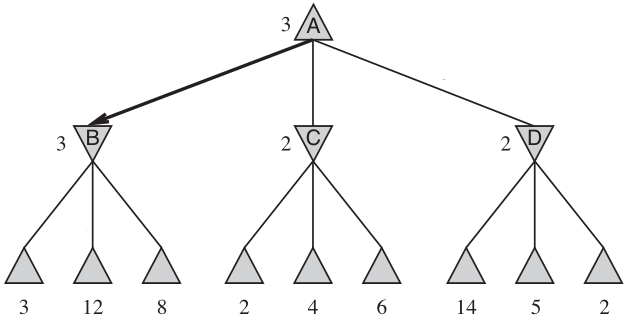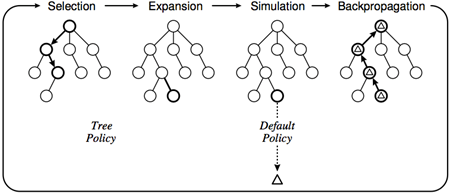# 对抗搜索简介¶

## 前置知识¶

• 具有最基本的程序设计思想，对于深度优先搜素、广度优先搜索等最基本的搜索算法有着一定的了解。
• 在这篇文章中 Snake Go 会作为例子出现，其具体规则请参照 Saiblo 游戏链接

## 概述¶

• 游戏有且仅有两个玩家参与，分轮进行，双方轮流做出决策。
• (Fair) 双方均知道游戏的一切完整信息。
• (Markov) 任意一个游戏者在某一轮可以做出的决策只与当前轮的游戏状态有关，与之前或者之后的决策无关。
• (Finite) 游戏一定会在有限轮内结束。
• (Zero-Sum) 游戏结束时一定有一方胜利，另一方失败，不存在平局。
• 我们称某方胜利了，当且仅当在某一轮中轮到对方行动，但对方没有合法的决策。

## SG 搜索¶

$s$ 表示游戏的一个状态，它通常是 $(\mathrm{turn}, \mathrm{player}, \mathrm{context})$ 的三元组，即游戏的轮数、该轮应当行动的玩家以及游戏的其他信息。

$\mathrm{action}(s) = \{a_1, a_2, \cdots, a_m\}$ 是一个集合，给出了 $s$ 状态下所有可能的决策。如果 $\mathrm{action}(s) = \varnothing$ 则说明 $s$ 是终态，用 $s \in V_T$ 表示，此时游戏已经结束并且 $\mathrm{player}$ 失败了。

$\delta(s, a)=s'$ 是转移函数，它表示在状态 $s$ 采取决策 $a$ 后转移到的状态为 $s'$

1. 如果 $s \in V_T$，则 $s$ 是先手必败的，否则考虑 $s$ 能够转移到的所有集合： $$\mathrm{ch}[s] = \{s' \mid \delta(s, a) = s', \forall a \in \mathrm{action}(s)\}$$

2. 如果 $\forall t, t\in \mathrm{ch}[s]$$t$ 是先手必胜的，那么 $s$ 是先手必败的。

3. 如果 $\exists t, t\in \mathrm{ch}[s]$$t$ 是先手必败的，那么 $s$ 是先手必胜的。

### 例子：Nim 游戏¶

$n$ 堆石子，每堆有 $a_i > 0$ 个石子。双方轮流取一堆石子中任意数量的石子，但不能不取。不能行动者失败。

• 基础：$(0,0,0)$ 是先手必败状态。
• $(1,0,0),(0,1,0),(0,0,1),(0,2,0)$ 是先手必胜状态，因为可以转移到 $(0,0,0)$
• $(1,1,0),(0,1,1),(1,0,1)$ 是先手必败状态，因为只能转移到上一行的状态中。
• $(1,1,1),(1,2,0),(0,2,1)$ 是先手必胜状态，因为可以转移到上一行的状态中。
• $(1,2,1)$ 是先手必胜状态，因为可以转移到 $(1,0,1)$

  1 2 3 4 5 6 7 8 9 10 int sg(int a[], int n) { int ret = 0 for (int i = 0; i < n; ++i) { if (a[i] > 0) for (int j = 1; j < a[i] + 1; ++j) b[] = a[], b[i] = a[i] - j ret |= !sg(b, n) } return ret } 

## Min-Max 搜索¶

SG 搜索固然确定地得到正确答案，但是时间复杂度与状态数相关。对于更复杂的问题，由于各种限制我们往往不能遍历所有可能状态，因此我们或许可以通过牺牲正确性来换取可行性。

1. 设根节点 $S_0$ 的深度为 $0$，每进行一次搜索深度加一。
2. 对于叶节点 $l$，其估值 $w_l = f(l)$
3. 对于深度为 $d$ 的非叶节点 $n$
• 如果 $d$ 是偶数，则 $w_n = \max_{s\in \mathrm{ch}[n]}(w_s)$，即其所有孩子估值的最大值。
• 如果 $d$ 是奇数，则 $w_n = \min_{s\in \mathrm{ch}[n]}(w_s)$，即其所有孩子估值的最小值。## Monte-Carlo 树搜索¶

1. 选择
• $S_0$ 出发，选择一个可拓展的节点 $s$ 并随机的拓展其一个子节点。一个节点是可拓展的，当且仅当其对应的状态 $s$ 不是终态并且存在 $t\in \mathrm{ch}[s]$，且 $t$ 不在搜索树中。
2. 拓展
• 假设选择拓展 $t\in \mathrm{ch}[s]$, 那么在搜索树中添加一个状态为 $t$ 的节点，并设其为 $s$ 的子节点。
3. 模拟
• $t$ 为初态实施默认策略，计算默认策略的权值增量 $\Delta$
• 如果默认策略中相对于 $S_0$ 胜利，则 $\Delta = 1$，否则 $\Delta = 0$
4. 回溯
• $S_0$$t$ 的路径上所有节点为 $s_1, s_2, \cdots, t$ 依次更新其状态：
• 如果节点 $N$ 的深度与 $L$ 同奇偶，更新为 $(s, w, n)\to (s, w + \Delta, n + 1)$
• 如果节点 $N$ 的深度与 $L$ 不同奇偶，更新为 $(s, w, n)\to (s, w + 1 - \Delta, n + 1)$
• 如果时间足够，回到“选择”部分重复。### 置信上界树¶

• 在 Min-Max 搜索中，我们最终只会选择每层节点中最大或最小的节点进行决策，因此对于其“平庸”的兄弟并不关心，而朴素的算法是进行等概率的随机选择。
• 我们需要考虑模拟次数对于估值的影响，一个模拟 1 次胜率为 0% 的节点，不一定比模拟 100 次胜率为 10% 的节点差。

UCB 的想法是改善选择拓展节点的方案，这个方案应该具有两方面的单调性：相同模拟次数下，胜率高的节点应该更多的被访问；相同胜率下，模拟次数少的估值应当更多的被访问。

I(s) = \frac{w}{n} + C\sqrt{\frac{2\ln(N)}{n}}

  1 2 3 4 5 6 7 8 9 10 11 12 13 14 15 16 17 18 19 20 21 22 23 24 25 26 27 28 29 30 31 32 33 34 35 36 37 38 39 40 41 42 43 44 45 46 47 48 49 50 51 52 53 54 55 56 57 """ UCT_search algorithm """ C = some configurable value def getinfo(v) # 输入一个节点 v，返回节点对应的 (s, w, n) def update(v, dw) # 更新节点的 v 对应的状态 w = w + dw, n = n + 1 def UCT_search(S0): root = create_tree(S0) while 时间足够: v = select(v0) dw = default_policy(v) back_propagate(v, dw) return best_child(root) def select(u): while u 不是终止节点: if u 是可拓展的: return expand(u) else: u = best_child(u) return u def expand(u): s, _, _ = getinfo(u) for t in ch[s]: if t 未被加入树中: return t def best_child(u): _, _, N = getinfo(u) I_max = -inf r = None for v in u.children: _, w, n = getinfo(v) I = w / n + sqrt(2 * ln(N) / n) if I > I_max: I_max = I r = v return r def default_policy(u): s, _, _ = getinfo(u) while s 不是终态: a = 随机选取一个行动 s = delta(s, a) if u 与 root 深度同奇偶: return 1 else: return 0 def back_propagate(u, dw): while u != root: update(u, dw) dw = 1 - dw u = u.parent 

• 人工智能导论